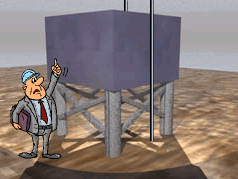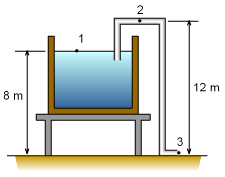Ch 7. Incompressible and Inviscid Flow Multimedia Engineering Fluids Bernoulli'sEquation FlowMeasurements Superpositionof Flows Flow aroundCylinder
 Chapter 1. Basics 2. Fluid Statics 3. Kinematics 4. Laws (Integral) 5. Laws (Diff.) 6. Modeling/Similitude 7. Inviscid 8. Viscous 9. External Flow 10. Open-Channel Appendix Basic Math Units Basic Equations Water/Air Tables Sections Search eBooks Dynamics Fluids Math Mechanics Statics Thermodynamics Author(s): Chean Chin Ngo Kurt Gramoll ©Kurt GramollFLUID MECHANICS - CASE STUDY IntroductionWater Reservoir and Siphon Pipe Engineer Steve has been sent to inspect a water reservoir with a pipe acting as a water siphon. He would like to find out if the pipe is installed properly so that cavitation will not occur inside the pipe. If cavitation does occur, what should he recommend to change the system? The dimensions of the system are given in the figure. Questions Determine the pressure at point 2 for the current system. Find the allowable height of the pipe such that cavitation will not occur. ApproachProblem Description Assume that points 1, 2 and 3 lie on a streamline, and Bernoulli's equation is applicable. For constant diameter pipe, the velocity at points 2 and 3 is the same. Cavitation occurs at a vapor pressure of pv = 2.34 kPa (abs). Assume the tank is large so that V1 = 0. Assume the outlet of the pipe is acting like a free jet, i.e., p3 = 0. Assume the density of water is 1000 kg/m3.

Practice Homework and Test problems now available in the 'Eng Fluids' mobile app
Includes over 250 problems with complete detailed solutions.
Available now at the Google Play Store and Apple App Store.